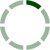### Sort by Date ▼ / Top Rated

End of Year vs Beginning of Year
Looking for clarity. We input \$352,541 for the 2006 total revenue column, however it is listed under the 01/2007 row of the forecast document. Is it because \$352,541 represents the total revenue for the entire 2006? The 01/2007 row would then represent the end of 2006.
0
error
Hi as someone mentioned before, there is an error for the eps for 2007 which should be 3.34.
In order to get full credit do I put the incorrect number from the video or do I put the correct one from the pdf?
0
Total Debt
Why is there a total debt cell (\$34,778) but then when we calculate the total debt/total capitalization, and the total debt/EBITDA the numerator is total debt + preferred stock + minority interest?